# 13 Essential Algorithms For Programmers

Algorithms are everywhere in programming, and with good reason. They provide a set of instructions for solving all kinds of software problems. Algorithms can make life easier for developers by arming them with a range of general problem-solving techniques.

There are thousands of programming algorithms out there today, so good software developers and engineers need to know what is available and when it is most appropriate to use them. A good algorithm will find the most efficient way to perform a function or solve a problem, both in terms of speed and by minimizing the use of computer memory.

Many problems can be resolved by starting with some of the most popular algorithms according to the function required. For example, sorting algorithms are instructions for arranging the items of an array or list into a particular order, while searching algorithms are used to find and retrieve an element from wherever it is stored in a data structure. Here are 11 algorithms that we think every programmer should know about:

## Sorting Algorithms

Sorting raw data sets is a simple but crucial step in computer/data science, and is increasingly important in the age of big data. Sorting typically involves finding numerical or alphabetical orders (ascending or descending).

• Insertion Sort: This orders an array one step at a time, building a sorted list by reviewing each unordered item and moving it into a suitable place. It is often compared to how we typically order a set of cards after being dealt a random hand (e.g. moving lower numbers to the left and kings/queens to the right until they are in ascending order). It is a fast and effective algorithm on smaller data sets.
• Selection Sort: This is a variation on the above. With selection sorts, the data is divided into two sections of ‘sorted’ and ‘unsorted’. The algorithm scans the unsorted section to find the smallest value and moves it to the sorted section (on the left of the list). It then repeats this process, gradually building a sorted array in ascending order. Again, this is more suitable for small data sets.
• Bubble Sort: This works by sweeping through every item in a list and swapping adjacent items if they are in the wrong order. The process is repeated until no elements in the list are left out of place. For example, in a randomized numerical sequence that you want to be arranged into ascending order, the algorithm will pass over the set several times until all the numbers fit the sequence.

As with Insertion, this is best used where the data structure is relatively small or is already partially sorted, as otherwise, it can be a time-consuming process.

• Quick Sort: This is known as a ‘divide and conquers’ algorithm as it continually breaks down a data structure into sub-lists to solve the sorting problem. In this case, one element becomes a ‘pivot’ and all the other elements are partitioned according to whether they are greater or smaller than the pivot value. Then the process is repeated in these sub-lists until each only has one item, by which time the whole series will be ordered.

• Merge Sort: This is another divide and conquer instruction that continually divides the data structure into halves until each sub-list has only one or two items. These are then put in the order required, and finally, all the sub-lists are merged together in the correct order.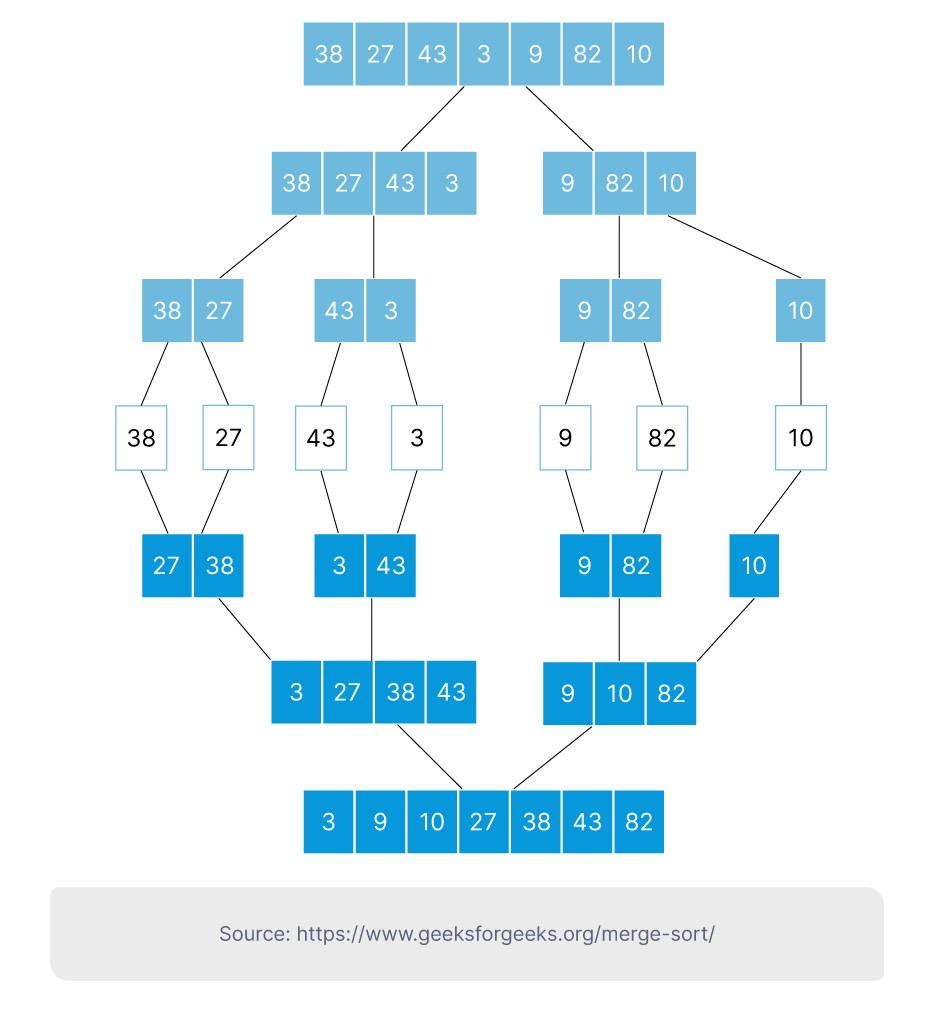## Searching Algorithms

Searching is such a basic IT function, but so important to get right when programming. It could involve searching for something within an internal database or trawling virtual spaces for a specific piece of information, and there are two standard approaches used today.

• Linear Search: This is a simple algorithm used to find a specific element or piece of information in an unsorted data set. It uses a sequential search format. For example, if you need to retrieve one mobile phone number from a huge database of random people, a linear search algorithm will go through each one sequentially until it finds the relevant item.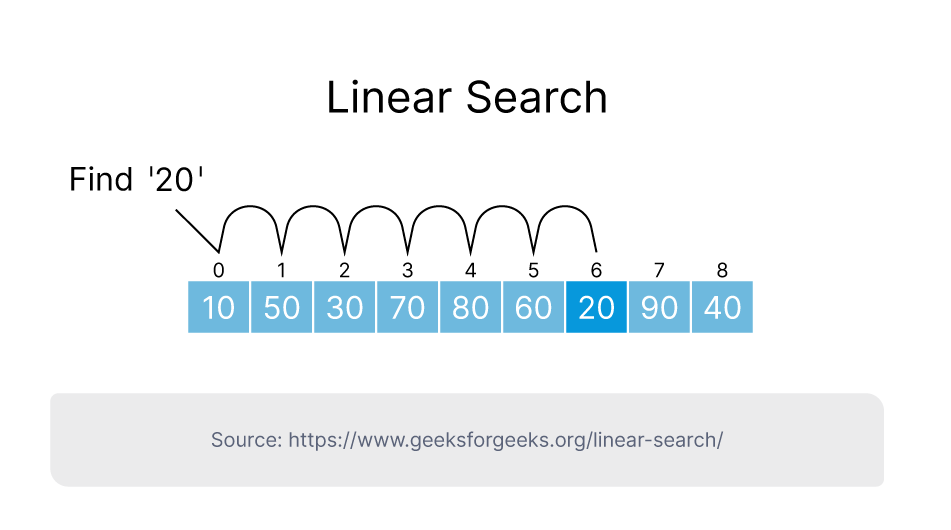• Binary Search: This uses a different approach, searching data structures by intervals rather than sequentially. It can be a more efficient method when used on sorted array data structures, as there is no need to scan the whole series.
For example, in a long series of ascending numbers, the binary search will begin with the middle element - if this is not a match and is below the number required, then it will find the middle item of the half-list above that point (if the middle item was above the number required, it would search the half below this point). This process will continue until it finds the item or there are no sub-lists left, meaning the target item is not there.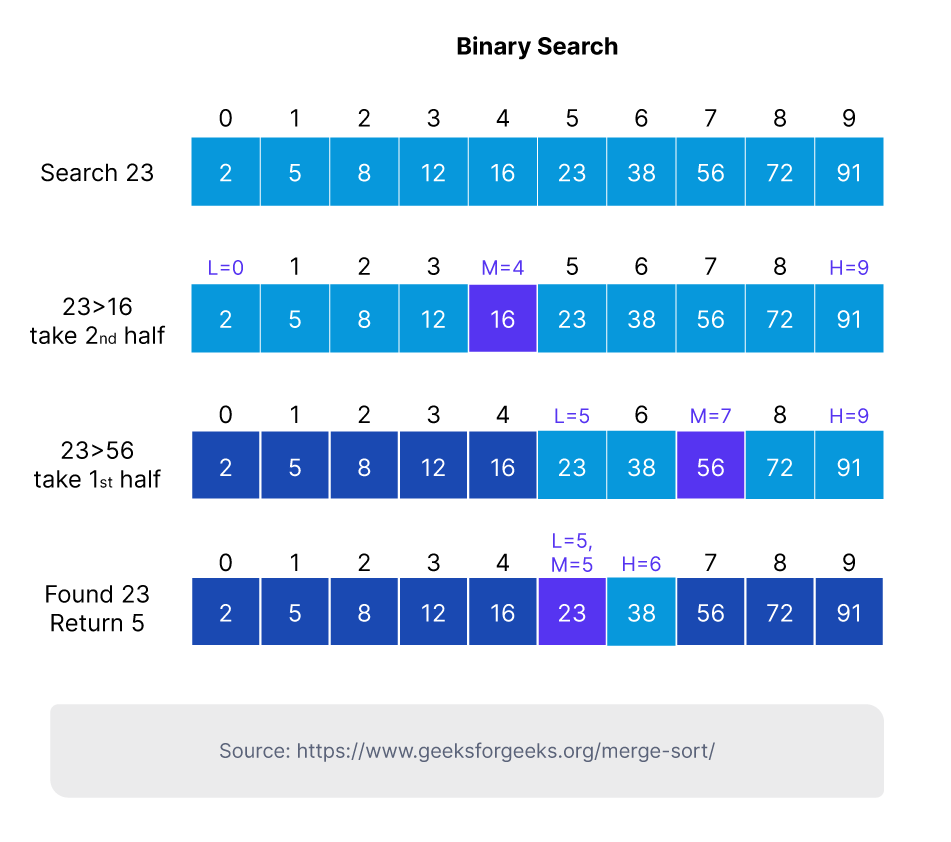## Graph Algorithms

Graphs are a vital tool in data visualization, commonly used to represent data items as interconnected ‘nodes’ in a network. These nodes could represent individual users of a social media platform or locations in a city. Basically, if you have a set of objects that are related to each other, then you can most likely represent them using a graph.

• Breadth-First Search (BFS): This is used to traverse tree or graph structures by starting at a single ‘root’ and exploring all neighboring nodes. It will then move on to the next nearest node that hasn’t yet been explored and repeat the process at the next level, continuing in this way until all nodes have been analyzed. It is a useful method of finding the shortest path through the nodes of a graph structure.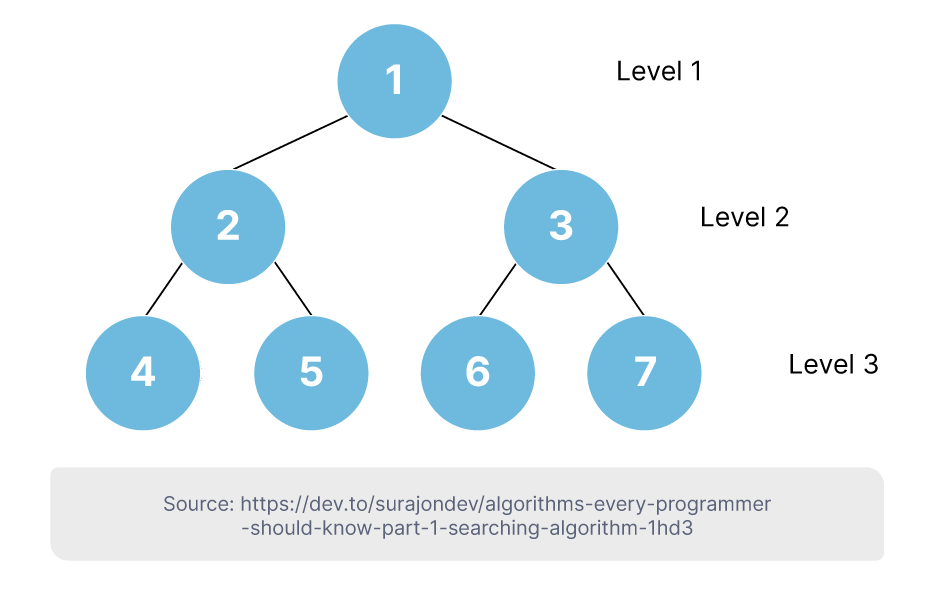• Depth-First Search (DFS): Rather than spreading out from the root one layer at a time as with BFS, the DFS algorithm will select a root node and then explore as far as possible along one branch that leads from it (and all of its sub-branches). It then returns along the branch to the root and starts the process again along another branch. It is often a more suitable option when the target node is a long way from the source.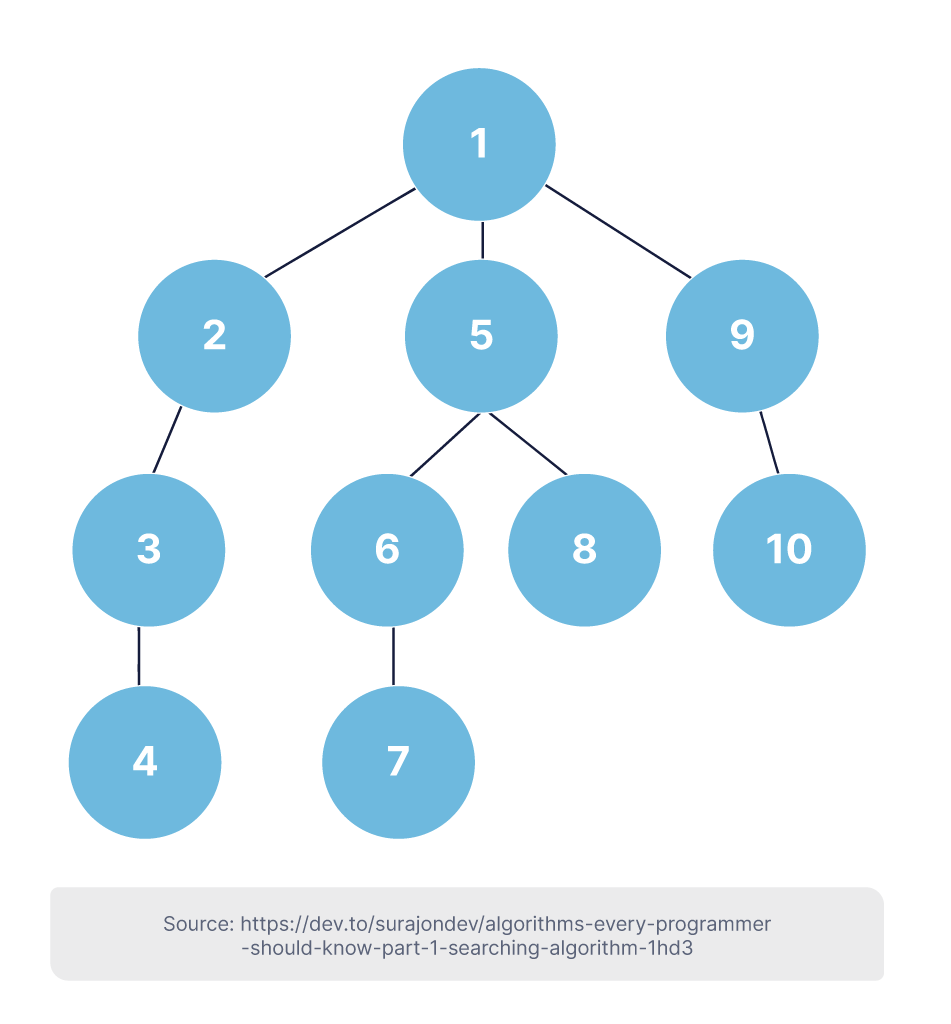• Dijkstra (Shortest-Path): This finds the shortest path between nodes in a graph structure. The algorithm, named after Dutch computer scientist Edsger W. Dijkstra, maps a tree of shortest paths from the starting node to every other connected node. Perhaps the most well-known application of this algorithm is Google maps, which quickly calculates the fastest route between any two points on a map (and updates this continually).

• Kruskal/Prim: These are two ‘greedy’ algorithms used to find the minimum spanning tree (MST) of a weighted graph structure. The MST means the minimum weight of the edges used to connect all the nodes (or vertices) in a graph structure. The Kruskal algorithm adds edges with the lowest weight in a structure until they connect up to form an MST. The Prim algorithm finds an MST by starting with a random vertex and building out to the next vertex via the lowest weighted edges.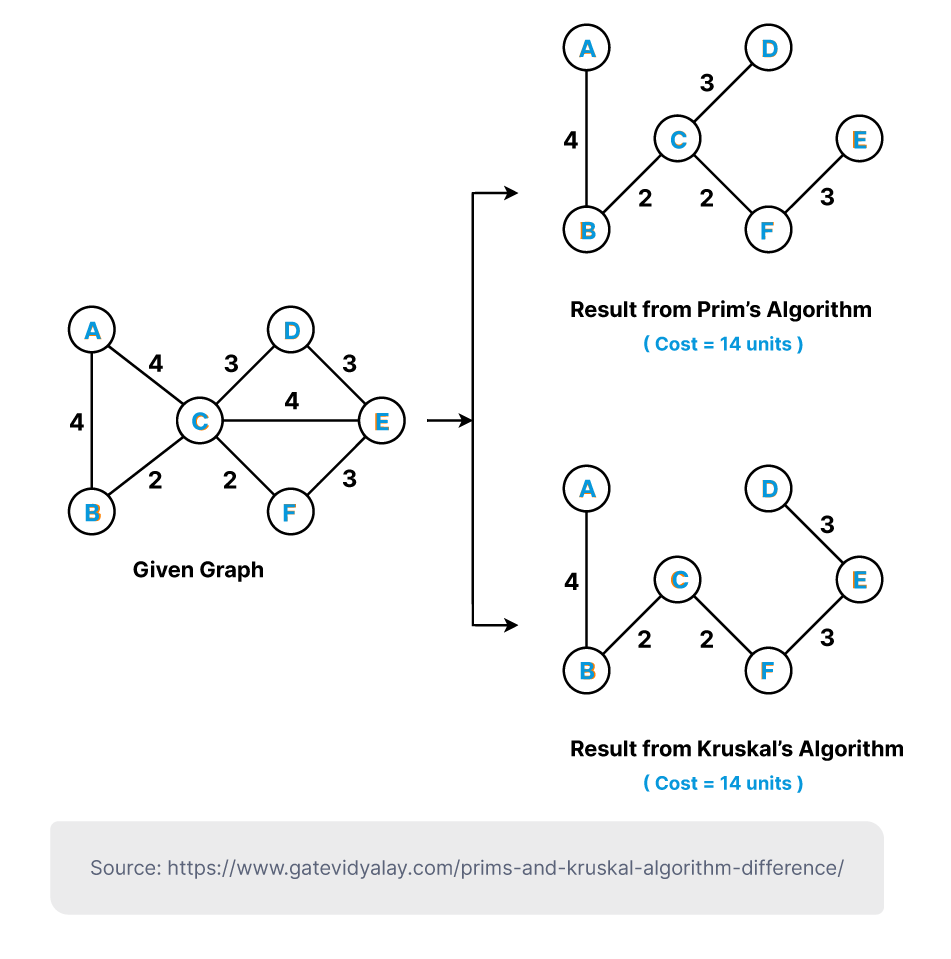## Others

• Hashing: This is increasingly common as we handle data structures at a previously unimaginable scale and become increasingly concerned with security. Hashing converts data of arbitrary length into a fixed length (the ‘hash value’), which provides a shorter summary of the original data. One common use for hash tables is for the encryption of keys or messages.

• Randomized: These algorithms necessarily include a degree of randomness as part of their logic, and are usually used with large volumes of data that would be difficult to process. The random element could be the expected time needed to find the correct solution (i.e. Las Vegas) or the probability that a particular result is correct after the algorithm is run over a set time (e.g. Monte Carlo).

--

If you want to stay up to date with all the new content we publish on our blog, share your email and hit the subscribe button.

Also, feel free to browse through the other sections of the blog where you can find many other amazing articles on: Programming, IT, Outsourcing, and even Management.